Angle Relationships in Triangles and Transversals | Math Grade 6, 7, & 8
1%
It was processed successfully!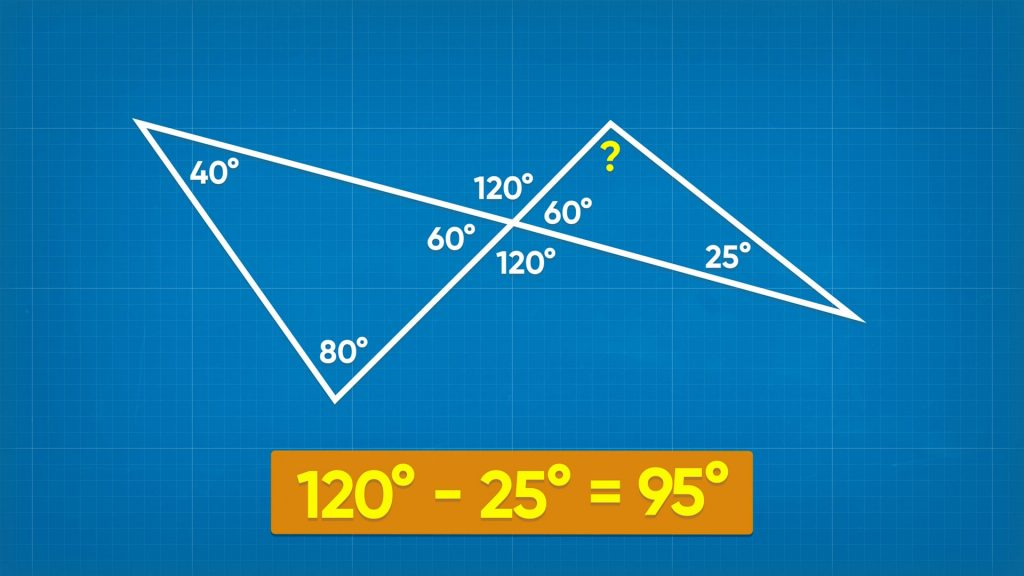WHAT ARE ANGLE RELATIONSHIPS?

The angles in a triangle have a special relationship. This means that if you know the measures of two of the angles, you can predict the measure of the third. There are also special relationships between the angles made when a line intersects a pair of parallel lines.

To better understand angle relationships…

WHAT ARE ANGLE RELATIONSHIPS?. The angles in a triangle have a special relationship. This means that if you know the measures of two of the angles, you can predict the measure of the third. There are also special relationships between the angles made when a line intersects a pair of parallel lines. To better understand angle relationships…

## LET’S BREAK IT DOWN!

### Review angle terminology.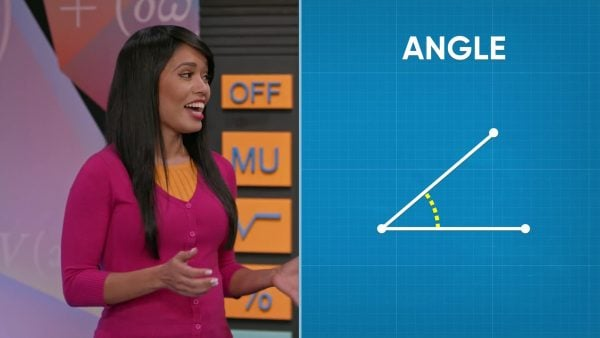An angle measures the space between two rays, lines, or line segments. Angles are measured in degrees. A full circle measures 360°, a half circle 180°, and a quarter circle 90°. Try this one yourself: What is the measure of the angle of [ggfrac]3/4[/ggfrac] of a circle?

Review angle terminology. An angle measures the space between two rays, lines, or line segments. Angles are measured in degrees. A full circle measures 360°, a half circle 180°, and a quarter circle 90°. Try this one yourself: What is the measure of the angle of [ggfrac]3/4[/ggfrac] of a circle?

### The sum of angles that form a line is 180°.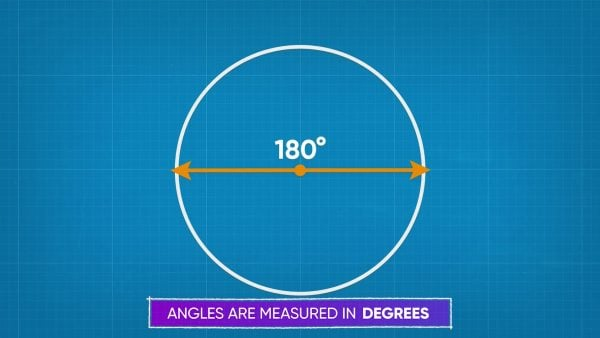Take a straight line and intersect that line with another line. This makes two angles. Imagine that one of those angles measures 90°. What is the measure of the other angle? You know that both angles must add to 180°, since a straight line shows a 180° angle. So the other angle measures 180 – 90 = 90°. What if the first angle measures 60°? Then the other angle measures 180 – 60 = 120°. Two angles that add to 180° are called supplementary angles. Try this one yourself: One angle measures 75°. What is the measure of the second angle that forms a line with the first angle?

The sum of angles that form a line is 180°. Take a straight line and intersect that line with another line. This makes two angles. Imagine that one of those angles measures 90°. What is the measure of the other angle? You know that both angles must add to 180°, since a straight line shows a 180° angle. So the other angle measures 180 – 90 = 90°. What if the first angle measures 60°? Then the other angle measures 180 – 60 = 120°. Two angles that add to 180° are called supplementary angles. Try this one yourself: One angle measures 75°. What is the measure of the second angle that forms a line with the first angle?

### The sum of the angles in a triangle is 180°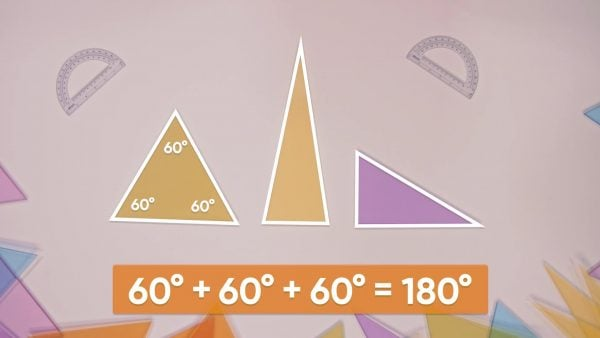Imagine you have 3 copies of the same triangle. The angles are labeled A, B, and C. Rotate the triangles to put one of each copy of each angle together. It forms a straight line! Is the sum of the measures of the angles in a triangle 180°? To test this, Emily and Amari measure the angles in a set of three triangles. Triangle 1 has angles that measure 60°, 60°, and 60°. That is 180° in all. Triangle 2 has angles that measure 80°, 80°, and 20°. That is 180° in all. Triangle 3 has angles that measure 80°, 30°, and 60°. That's 180° as well! The measures of the 3 angles of a triangle always add up to 180°. That means, if you know two angles, you can find the measure of the third. A triangle has angles that measure 40° and 60°. What is the measure of the third angle? 180 – 40 – 60 = 80°. The last angle measures 80°. Try this one yourself: A triangle has angles that measure 70° and 45°. What is the measure of the third angle?

The sum of the angles in a triangle is 180° Imagine you have 3 copies of the same triangle. The angles are labeled A, B, and C. Rotate the triangles to put one of each copy of each angle together. It forms a straight line! Is the sum of the measures of the angles in a triangle 180°? To test this, Emily and Amari measure the angles in a set of three triangles. Triangle 1 has angles that measure 60°, 60°, and 60°. That is 180° in all. Triangle 2 has angles that measure 80°, 80°, and 20°. That is 180° in all. Triangle 3 has angles that measure 80°, 30°, and 60°. That's 180° as well! The measures of the 3 angles of a triangle always add up to 180°. That means, if you know two angles, you can find the measure of the third. A triangle has angles that measure 40° and 60°. What is the measure of the third angle? 180 – 40 – 60 = 80°. The last angle measures 80°. Try this one yourself: A triangle has angles that measure 70° and 45°. What is the measure of the third angle?

### Vertical angles are always equal.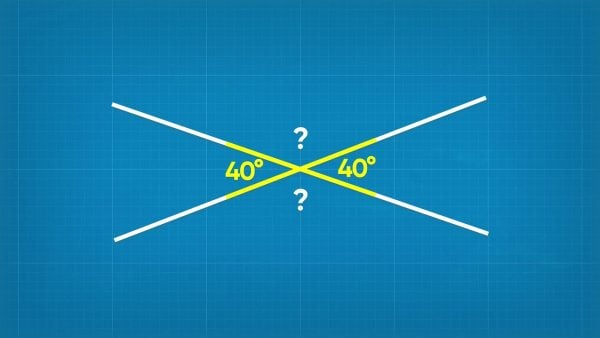Imagine that you've drawn two lines that intersect. The measure of the angles that are opposite each other are always equal. These angles are called vertical angles. So, if you know one angle, you automatically know the other. If two lines cross, forming one angle that is 40°, the angle opposite that angle is also 40°.

Vertical angles are always equal. Imagine that you've drawn two lines that intersect. The measure of the angles that are opposite each other are always equal. These angles are called vertical angles. So, if you know one angle, you automatically know the other. If two lines cross, forming one angle that is 40°, the angle opposite that angle is also 40°.

### A transversal is a line that crosses two parallel lines.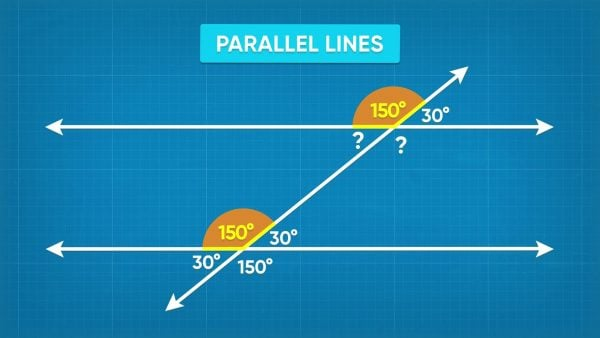When a transversal crosses two parallel lines, the angles formed have special relationships. First, let's talk about some vocabulary. Angles that are in the same position on both intersections are called corresponding angles. Corresponding angles are always equal. Angles that are both on the inside of the parallel lines are called interior angles. Interior angles add to 180°. Angles that are both on the outside of the parallel lines are called exterior angles. Exterior angles add to 180°. Angles that are across from each other on different lines are called alternate angles. Alternate interior angles are always equal. Alternate exterior angles are also always equal! Using all of the relationships you've seen so far, knowing just one angle can help you find every angle formed by the intersecting lines. Try this one yourself: The measure of an angle formed by a transversal is 120°. What is the measure of the corresponding angle on the other line? What is the measure of the alternate exterior angle to the 120° angle?

A transversal is a line that crosses two parallel lines. When a transversal crosses two parallel lines, the angles formed have special relationships. First, let's talk about some vocabulary. Angles that are in the same position on both intersections are called corresponding angles. Corresponding angles are always equal. Angles that are both on the inside of the parallel lines are called interior angles. Interior angles add to 180°. Angles that are both on the outside of the parallel lines are called exterior angles. Exterior angles add to 180°. Angles that are across from each other on different lines are called alternate angles. Alternate interior angles are always equal. Alternate exterior angles are also always equal! Using all of the relationships you've seen so far, knowing just one angle can help you find every angle formed by the intersecting lines. Try this one yourself: The measure of an angle formed by a transversal is 120°. What is the measure of the corresponding angle on the other line? What is the measure of the alternate exterior angle to the 120° angle?

## VOCABULARY

Angle
An angle is formed by the intersection of two rays, lines, or line segments.
Degree
The unit used to measure angles.
Supplementary
Vertical Angles
When two lines intersect, they form 4 angles. Vertical angles are opposite each other in the intersection.
Parallel Lines
Line that, when continued infinitely, never cross.
Transversal
A line that crosses a pair of parallel lines.
Angles that are in the same position on both intersections of a transversal with two parallel lines.
Angles that are both on the inside of the parallel lines, formed by the transversal.
Angles that are both on the outside of the parallel lines, formed by the transversal.
Angles that are across from each other on different lines, where they intersect with the transversal.

## DISCUSSION QUESTIONS

### What are supplementary angles? How can you use supplementary angles to solve problems?

Supplementary angles have measures that sum to 180°. If I know one angle in a supplementary angle pair, I can subtract it from 180 to find the measure of the missing angle.

### What is the relationship between the angles in a triangle?

The measures of the angles in a triangle add to 180°.

### What are vertical angles? What is the relationship between vertical angles?

When two lines intersect, vertical angles are opposite each other. Vertical angles are congruent, so they have equal measure.

### What is a transversal?

A transversal is a line that intersects a pair of parallel lines.

### What are some of the relationships between the angles formed when a transversal intersects two parallel lines?

Corresponding angles are in the same position on both intersections; they are congruent. Interior angles are both on the inside of the parallel lines; they are supplementary. Exterior angles are both on the outside of the parallel lines; they are supplementary. Alternate angles are across from each other on different lines; alternate exterior and alternate interior angles are congruent.
X

## Success

We’ve sent you an email with instructions how to reset your password.
Ok